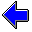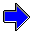Java Security Settings:
This web page employs Java, which requires specific security settings for correct operation.
If the applets on this page do not run correctly, consult the Virtual Chemistry Experiments FAQ
or the Physlet Physics web site for establishing the correct security settings.

# Calorimetry

## Specific Heat Capacity of Copper

### Concepts

Calorimeters are designed to be well-insulated, so no heat is gained from or lost to the surroundings. If no heating element is used to introduce heat in the system, the total heat transferred (q) for the entire calorimeter system must equal zero. The total heat can be split into heats for each component in the system.

Imagine an experiment in which a hot copper ball is dropped into a calorimeter containing water at room temperature. The copper ball will lose heat, which will be absorbed by the calorimeter and water. Because no heat enters or leaves the system the heat balance for this experiment is

0 = q = qCu + qcal + qw

In this case qCu < 0, because the copper ball will lose heat to the calorimeter and water. Similarly qcal > 0 and qw > 0, because both the calorimeter and the water will gain heat. In chemistry the thermodynamic sign convention is things entering the system a positive in value, and things leaving the system are negative in value.

The basic strategy in calorimetry is to use a temperature change and a heat capacity to determine a heat flow. In this experiment, all substances have the same final temperature (Tf), but not all substances have the same initial temperature. The copper ball is initially at temperature TCu while the calorimeter and water are initially at temperature Ti.

qCu = mCu sCu ( Tf - TCu )

qcal = Ccal
( Tf - Ti )

qw = mw sw
( Tf - Ti )

The heat capacity of the calorimeter must be obtained from a separate calibration experiment (for example, a heating element can be used to introduce a known amount of heat). The specific heat capacity of water is known (4.184 J oC-1), and the temperatures TCu, Ti, and Tf can be measured experimentally. The masses of the copper and water (mCu and mCu) can also be measured experimentally. The only unknown property in the above equations is the specific heat capacity of the copper.

 sCu = - (Ccal + mw sw) (Tf - Ti)mCu (Tf - TCu)

### Experiment

Objective:

• Determine the specific heat capacity of copper.

Approach:

• Part 1. Determine the heat capacity of the calorimeter using a metal of known specific heat capacity.
• Part 2. Determine the specific heat capacity of copper.

### Part 1

 Before the specific heat capacity of copper can be determined, it is necessary to know the heat capacity of the calorimeter. The value can be found by performing an experiment with a metal of known heat capacity. Iron will be used for this purpose: sFe = 0.450 J oC-1 g-1 In this experiment, the iron ball always starts at a temperature of 100.0 oC. Enter values for the masses of iron and water and perform the calorimetry experiment. Repeat the experiment several times using different parameters. You should always get the same value for Ccal, within experimental error. Mass of Iron g Mass of Water g

### Part 2

 Once the heat capacity of the calorimeter has been determined, it is possible to experimentally determine the specific heat capacity of copper. You will need to use the heat capacity of the calorimeter determined in Part 1 to perform the calculations for this part of the xperiment. In this experiment, the copper ball always starts at a temperature of 100.0 oC. Enter values for the masses of copper and water and perform the calorimetry experiment. Repeat the experiment several times using different parameters. You should always get the same value for sCu, within experimental error. Mass of Copper g Mass of Water gSpecific Heat Capacity of Ethanol                     Heat of Solution of Ammonium NitrateCalorimetry Home PageVirtual Chemistry Home Page

SpecificHeatCapacityOfCopper.html version 2.1
© 2000-2014 David N. Blauch